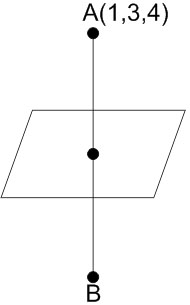#### Directions : Question are Assertion- Reason type questions. Each of these questions contains two statements. Statement-1 (Assertion) and Statement-2 (Reason). Each of these questions also has four alternative choices,only one of which is the correct answer. You have to select the correct choice:Statement-1 : The point(3, 1, 6) is the mirror image of the point(1, 3, 4) in the planeStatement-2 : The planebisects the line segment joining(3, 1, 6) and(1, 3, 4). Option 1) Statement -1 is true, statement -2 is true ; statement -2 is a correct explanation of statement-1. Option 2) Statement -1 is true, statement -2 is true ; statement -2 is not a correct explanation of statement-1. Option 3) Statement -1 is true, statement -2 is false. Option 4) Statement -1 is false, statement -2 is true.As we learnt in

Image of a point -

Letbe the image of pointin the planewill be given by the formula-Statement 1 is true as well.

But it is not necessary that if a plane bisects line joining A and B, that B is the image of A.

Option 1)

Statement -1 is true, statement -2 is true ; statement -2 is a correct explanation of statement-1.

Incorrect option

Option 2)

Statement -1 is true, statement -2 is true ; statement -2 is not a correct explanation of statement-1.

Correct option

Option 3)

Statement -1 is true, statement -2 is false.

Incorrect option

Option 4)

Statement -1 is false, statement -2 is true.

Incorrect option

##### Posted by